Publicité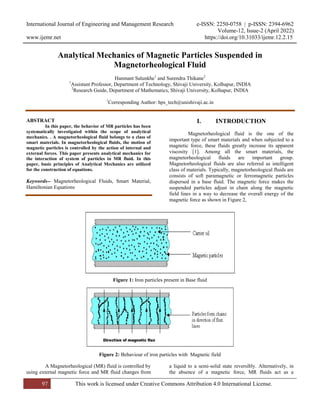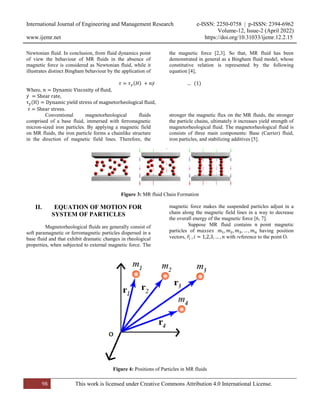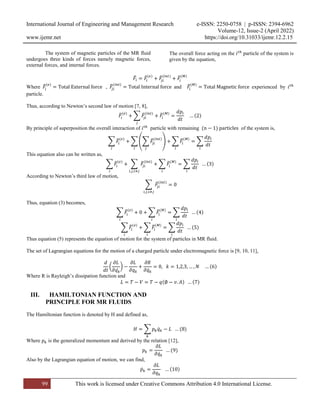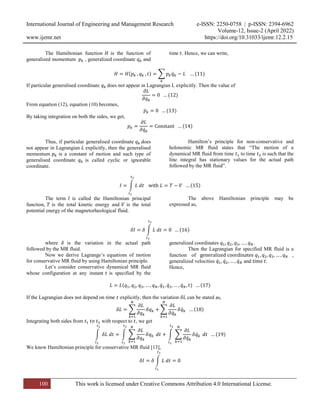Publicité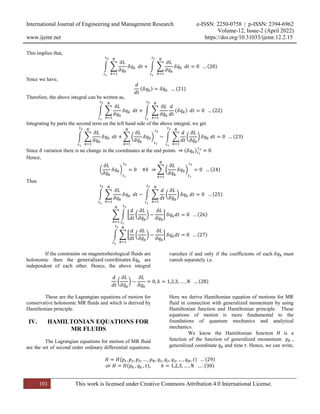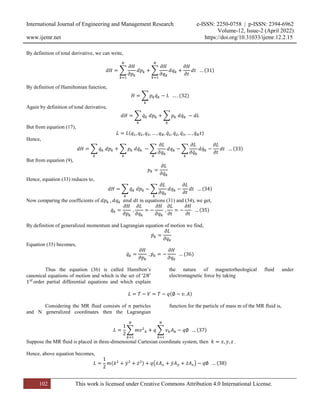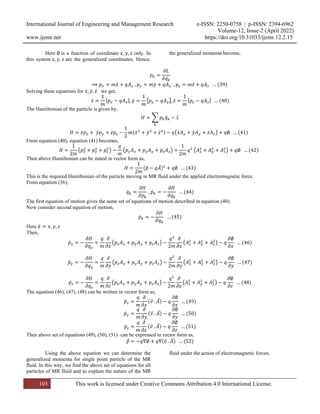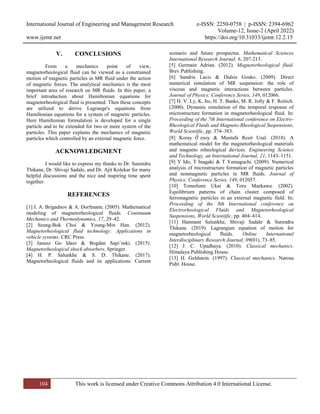Prochain SlideShare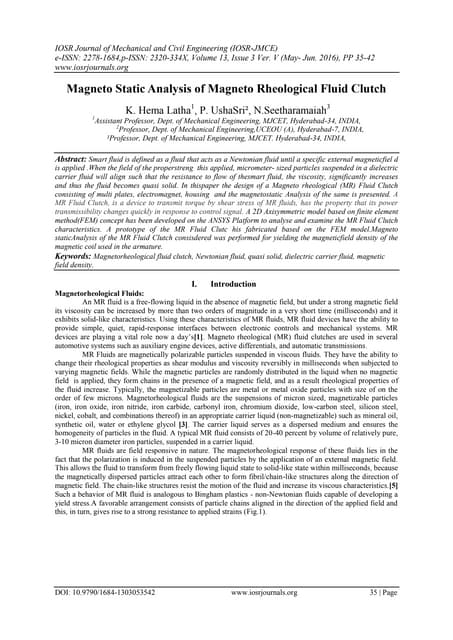F1303053542
Chargement dans ... 3
1 sur 8
Publicité

### Analytical Mechanics of Magnetic Particles Suspended in Magnetorheological Fluid

1. International Journal of Engineering and Management Research e-ISSN: 2250-0758 | p-ISSN: 2394-6962 Volume-12, Issue-2 (April 2022) www.ijemr.net https://doi.org/10.31033/ijemr.12.2.15 97 This work is licensed under Creative Commons Attribution 4.0 International License. Analytical Mechanics of Magnetic Particles Suspended in Magnetorheological Fluid Hanmant Salunkhe1 and Surendra Thikane2 1 Assistant Professor, Department of Technology, Shivaji University, Kolhapur, INDIA 2 Research Guide, Department of Mathematics, Shivaji University, Kolhapur, INDIA 1 Corresponding Author: hps_tech@unishivaji.ac.in ABSTRACT In this paper, the behavior of MR particles has been systematically investigated within the scope of analytical mechanics. . A magnetorheological fluid belongs to a class of smart materials. In magnetorheological fluids, the motion of magnetic particles is controlled by the action of internal and external forces. This paper presents analytical mechanics for the interaction of system of particles in MR fluid. In this paper, basic principles of Analytical Mechanics are utilized for the construction of equations. Keywords-- Magnetorheological Fluids, Smart Material, Hamiltonian Equations I. INTRODUCTION Magnetorheological fluid is the one of the important type of smart materials and when subjected to a magnetic force, these fluids greatly increase its apparent viscosity . Among all the smart materials, the magnetorheological fluids are important group. Magnetorheological fluids are also referred as intelligent class of materials. Typically, magnetorheological fluids are consists of soft paramagnetic or ferromagnetic particles dispersed in a base fluid. The magnetic force makes the suspended particles adjust in chain along the magnetic field lines in a way to decrease the overall energy of the magnetic force as shown in Figure 2, Figure 1: Iron particles present in Base fluid Figure 2: Behaviour of iron particles with Magnetic field A Magnetorheological (MR) fluid is controlled by using external magnetic force and MR fluid changes from a liquid to a semi-solid state reversibly. Alternatively, in the absence of a magnetic force, MR fluids act as a
2. International Journal of Engineering and Management Research e-ISSN: 2250-0758 | p-ISSN: 2394-6962 Volume-12, Issue-2 (April 2022) www.ijemr.net https://doi.org/10.31033/ijemr.12.2.15 98 This work is licensed under Creative Commons Attribution 4.0 International License. Newtonian fluid. In conclusion, from fluid dynamics point of view the behaviour of MR fluids in the absence of magnetic force is considered as Newtonian fluid, while it illustrates distinct Bingham behaviour by the application of the magnetic force [2,3]. So that, MR fluid has been demonstrated in general as a Bingham fluid model, whose constitutive relation is represented by the following equation , ( ) ̇ ( ) Where, ̇ ( ) , Conventional magnetorheological fluids comprised of a base fluid, immersed with ferromagnetic micron-sized iron particles. By applying a magnetic field on MR fluids, the iron particle forms a chainlike structure in the direction of magnetic field lines. Therefore, the stronger the magnetic flux on the MR fluids, the stronger the particle chains, ultimately it increases yield strength of magnetorheological fluid. The magnetorheological fluid is consists of three main components: Base (Carrier) fluid, iron particles, and stabilizing additives . Figure 3: MR fluid Chain Formation II. EQUATION OF MOTION FOR SYSTEM OF PARTICLES Magnetorheological fluids are generally consist of soft paramagnetic or ferromagnetic particles dispersed in a base fluid and that exhibit dramatic changes in rheological properties, when subjected to external magnetic force. The magnetic force makes the suspended particles adjust in a chain along the magnetic field lines in a way to decrease the overall energy of the magnetic force [6, 7]. Suppose MR fluid contains point magnetic particles of having position vectors, ̅ with reference to the point O. Figure 4: Positions of Particles in MR fluids
3. International Journal of Engineering and Management Research e-ISSN: 2250-0758 | p-ISSN: 2394-6962 Volume-12, Issue-2 (April 2022) www.ijemr.net https://doi.org/10.31033/ijemr.12.2.15 99 This work is licensed under Creative Commons Attribution 4.0 International License. The system of magnetic particles of the MR fluid undergoes three kinds of forces namely magnetic forces, external forces, and internal forces. The overall force acting on the particle of the system is given by the equation, ̅ ̅( ) ̅( ) ̅( ) Where ̅( ) , ̅( ) and ̅( ) experienced by particle. Thus, according to Newton’s second law of motion [7, 8], ̅( ) ∑ ̅( ) ̅( ) ( ) By principle of superposition the overall interaction of particle with remaining ( ) of the system is, ∑ ̅( ) ∑ (∑ ̅( ) ) ∑ ̅( ) ∑ This equation also can be written as, ∑ ̅( ) ∑ ̅( ) ∑ ̅( ) ∑ ( ) According to Newton’s third law of motion, ∑ ̅( ) Thus, equation (3) becomes, ∑ ̅( ) ∑ ̅( ) ∑ ( ) ∑ ̅( ) ∑ ̅( ) ∑ ( ) Thus equation (5) represents the equation of motion for the system of particles in MR fluid. The set of Lagrangian equations for the motion of a charged particle under electromagnetic force is [9, 10, 11], ( ̇ ) ̇ ( ) Where R is Rayleigh’s dissipation function and ( ) ( ) III. HAMILTONIAN FUNCTION AND PRINCIPLE FOR MR FLUIDS The Hamiltonian function is denoted by H and defined as, ∑ ̇ ( ) Where is the generalized momentum and derived by the relation , ̇ ( ) Also by the Lagrangian equation of motion, we can find, ̇ ( )
4. International Journal of Engineering and Management Research e-ISSN: 2250-0758 | p-ISSN: 2394-6962 Volume-12, Issue-2 (April 2022) www.ijemr.net https://doi.org/10.31033/ijemr.12.2.15 100 This work is licensed under Creative Commons Attribution 4.0 International License. The Hamiltonian function is the function of generalized momentum , generalized coordinate and time . Hence, we can write, ( ) ∑ ̇ ( ) If particular generalised coordinate does not appear in Lagrangian L explicitly. Then the value of ( ) From equation (12), equation (10) becomes, ̇ ( ) By taking integration on both the sides, we get, ̇ ( ) Thus, if particular generalised coordinate does not appear in Lagrangian explicitly, then the generalised momentum is a constant of motion and such type of generalised coordinate is called cyclic or ignorable coordinate. Hamilton’s principle for non-conservative and holonomic MR fluid states that “The motion of a dynamical MR fluid from time to time is such that the line integral has stationary values for the actual path followed by the MR fluid”. ∫ ( ) The term is called the Hamiltonian principal function, is the total kinetic energy and is the total potential energy of the magnetorheological fluid. The above Hamiltonian principle may be expressed as, ∫ ( ) where is the variation in the actual path followed by the MR fluid. Now we derive Lagrange’s equations of motion for conservative MR fluid by using Hamiltonian principle. Let’s consider conservative dynamical MR fluid whose configuration at any instant is specified by the generalized coordinates . Then the Lagrangian for specified MR fluid is a function of , generalized velocities ̇ ̇ ̇ and . Hence, ( ̇ ̇ ̇ ) ( ) If the Lagrangian does not depend on time explicitly, then the variation can be stated as, ∑ ∑ ̇ ̇ ( ) Integrating both sides from with respect to , we get ∫ ∫ ∑ ∫ ∑ ̇ ̇ ( ) We know Hamiltonian principle for conservative MR fluid , ∫
5. International Journal of Engineering and Management Research e-ISSN: 2250-0758 | p-ISSN: 2394-6962 Volume-12, Issue-2 (April 2022) www.ijemr.net https://doi.org/10.31033/ijemr.12.2.15 101 This work is licensed under Creative Commons Attribution 4.0 International License. This implies that, ∫ ∑ ∫ ∑ ̇ ̇ ( ) Since we have, ( ) ̇ ( ) Therefore, the above integral can be written as, ∫ ∑ ∫ ∑ ̇ ( ) ( ) Integrating by parts the second term on the left hand side of the above integral, we get ∫ ∑ ∑ ( ̇ ) ∫ ∑ ( ̇ ) ( ) Since variation there is no change in the coordinates at the end points ( ) . Hence, ( ̇ ) ∑ ( ̇ ) ( ) Thus ∫ ∑ ∫ ∑ ( ̇ ) ( ) ∑ ∫ [ ( ̇ ) ] ( ) ∫ ∑ [ ( ̇ ) ] ( ) If the constraints on magnetorheological fluids are holonomic then the are independent of each other. Hence, the above integral vanishes if and only if the coefficients of each must vanish separately i.e. ( ̇ ) ( ) These are the Lagrangian equations of motion for conservative holonomic MR fluids and which is derived by Hamiltonian principle. IV. HAMILTONIAN EQUATIONS FOR MR FLUIDS The Lagrangian equations for motion of MR fluid are the set of second order ordinary differential equations. Here we derive Hamiltonian equation of motions for MR fluid in connection with generalized momentum by using Hamiltonian function and Hamiltonian principle. These equations of motion is more fundamental to the foundations of quantum mechanics and analytical mechanics. We know the Hamiltonian function is a function of the function of generalized momentum , generalized coordinate and time . Hence, we can write, ( ) ( ) ( ) ( )
6. International Journal of Engineering and Management Research e-ISSN: 2250-0758 | p-ISSN: 2394-6962 Volume-12, Issue-2 (April 2022) www.ijemr.net https://doi.org/10.31033/ijemr.12.2.15 102 This work is licensed under Creative Commons Attribution 4.0 International License. By definition of total derivative, we can write, ∑ ∑ ( ) By definition of Hamiltonian function, ∑ ̇ ( ) Again by definition of total derivative, ∑ ̇ ∑ ̇ But from equation (17), ( ̇ ̇ ̇ ̇ ) Hence, ∑ ̇ ∑ ̇ ∑ ∑ ̇ ̇ ( ) But from equation (9), ̇ Hence, equation (33) reduces to, ∑ ̇ ∑ ( ) Now comparing the coefficients of in equations (31) and (34), we get, ̇ ( ) By definition of generalized momentum and Lagrangian equation of motion we find, ̇ Equation (35) becomes, ̇ ̇ ( ) Thus the equation (36) is called Hamilton’s canonical equations of motion and which is the set of order partial differential equations and which explain the nature of magnetorheological fluid under electromagnetic force by taking ( ) Considering the MR fluid consists of n particles and N generalized coordinates then the Lagrangian function for the particle of mass m of the MR fluid is, ∑ ∑ ( ) Suppose the MR fluid is placed in three-dimensional Cartesian coordinate system, then . Hence, above equation becomes, ( ̇ ̇ ̇ ) ( ̇ ̇ ̇ ) ( )
7. International Journal of Engineering and Management Research e-ISSN: 2250-0758 | p-ISSN: 2394-6962 Volume-12, Issue-2 (April 2022) www.ijemr.net https://doi.org/10.31033/ijemr.12.2.15 103 This work is licensed under Creative Commons Attribution 4.0 International License. Here is a function of coordinate only. In this system are the generalized coordinates. Hence, the generalized momenta become, ̇ ̇ ̇ ̇ ( ) Solving these equations for ̇ ̇ ̇ we get, ̇ [ ] ̇ [ ] ̇ [ ] ( ) The Hamiltonian of the particle is given by, ∑ ̇ ̇ ̇ ̇ ( ̇ ̇ ̇ ) ( ̇ ̇ ̇ ) ( ) From equation (40), equation (41) becomes, ( ) ( ) ( ) ( ) Then above Hamiltonian can be stated in vector form as, ( ̅ ̅) ( ) This is the required Hamiltonian of the particle moving in MR fluid under the applied electromagnetic force. From equation (36), ̇ ̇ ( ) The first equation of motion gives the same set of equations of motion described in equation (40). Now consider second equation of motion, ̇ ( ) Here Then, ̇ ( ) ( ) ( ) ̇ ( ) ( ) ( ) ̇ ( ) ( ) ( ) The equation (46), (47), (48) can be written in vector form as, ̇ ( ̅ ̅) ( ) ̇ ( ̅ ̅) ( ) ̇ ( ̅ ̅) ( ) Then above set of equations (49), (50), (51) can be expressed in vector form as, ̅̇ ( ̅ ̅) ( ) Using the above equation we can determine the generalized momenta for single point particle of the MR fluid. In this way, we find the above set of equations for all particles of MR fluid and to explain the nature of the MR fluid under the action of electromagnetic forces.